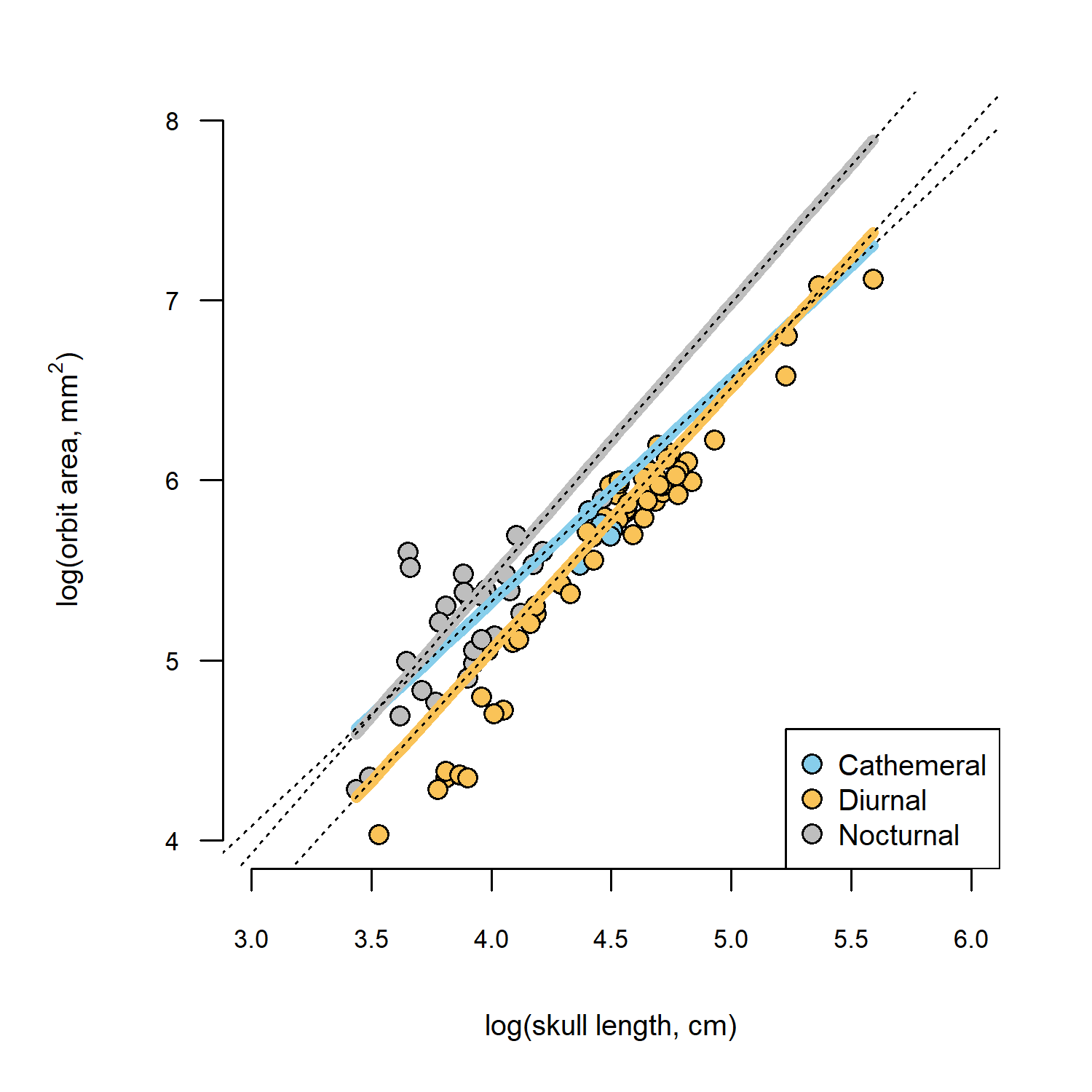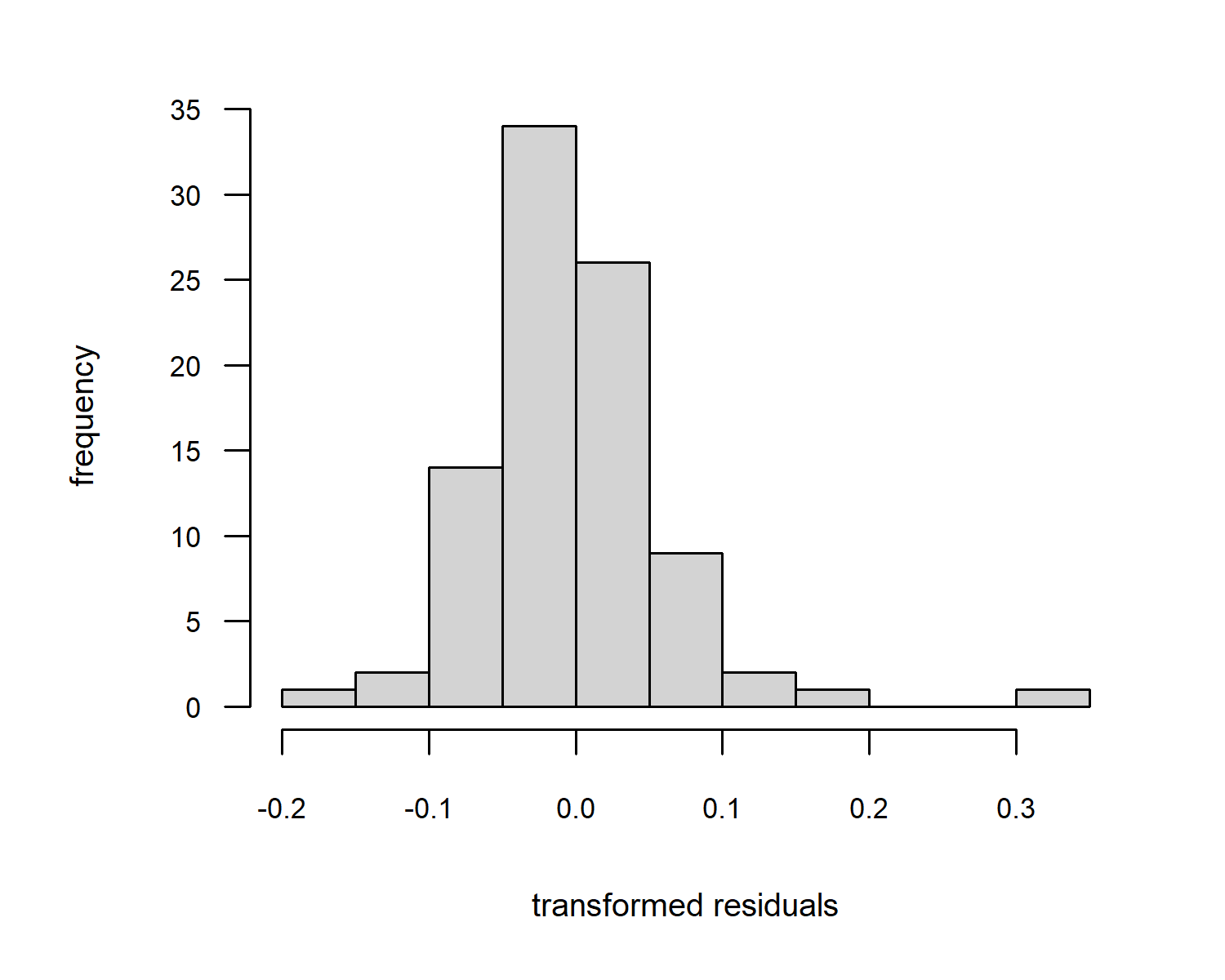### 1. Extracting slopes and 95% confidence intervals from phylogenetic ANCOVA

“In the example in Chapter 3 where you analyze Rich Kay and Chris Kirk’s data, I believe that I understand the output and that you also checked for interaction with activity pattern - no interaction and, therefore, similar slopes. The coefficients provide intercept differences between that of the cathemeral animals and those of the diurnal and nocturnal ones. These intercept differences make the calculation of the factor lines in Figure 3.3 possible if I’m understanding your walkthrough correctly. What I would like to know is whether it is possible to find the slopes and CIs for each group?”

This an excellent question & the answers are “yes” and “yes (but harder to plot them)”.

Let’s see how.

To begin with, I’ll load our packages and read our input data. Note that diel activity pattern in the files used in the creation of Chapter 3 from the book was mis-coded for Ateles. Though this did not qualitatively impact the analyses of the chapter, I’ve nonetheless corrected the error, and the updated data file (along with the tree) is now packaged with phytools (>=1.7-8). We’ll load this version instead of the one from the book, so our results may change slightly as a consequence.

library(phytools)
library(nlme)
data(primate.tree)
data(primate.data)

Next, I’ll build my hypothesized covariance structure of the residual error using corBrownian. If I had a different hypothesis, I could use the alternative function (e.g., corPagel) as appropriate.

spp<-rownames(primate.data)
corBM<-corBrownian(phy=primate.tree,form=~spp)

Now, I’m simply going to reiterate the analysis of Chapter 3 in the book, but this time allow different relationships between log(skull length) and log(orbit area). To do that, I write down the formula log(Orbit_area) ~ log(Skull_length) * Activity_pattern instead of log(Orbit_area) ~ log(Skull_length) + Activity_pattern from the book. In addition, to make our task a little bit easier, I’m going to add -1 to my formula and fit our ANCOVA model without an intercept term. This just has the effect of changing one of our factor levels into the intercept, but will make it a tiny more straightforward to compare their slopes!

primate.ancova<-gls(log(Orbit_area)~log(Skull_length)*
Activity_pattern-1,data=primate.data,
correlation=corBM)

Here is a print-out of our model object.

primate.ancova
## Generalized least squares fit by REML
##   Model: log(Orbit_area) ~ log(Skull_length) * Activity_pattern - 1
##   Data: primate.data
##   Log-restricted-likelihood: 48.91154
##
## Coefficients:
##                           log(Skull_length)                  Activity_patternCathemeral                     Activity_patternDiurnal
##                                   1.2459214                                   0.3387843                                  -0.7712570
##                   Activity_patternNocturnal   log(Skull_length):Activity_patternDiurnal log(Skull_length):Activity_patternNocturnal
##                                  -0.6716533                                   0.2110802                                   0.2855463
##
## Correlation Structure: corBrownian
##  Formula: ~spp
##  Parameter estimate(s):
## numeric(0)
## Degrees of freedom: 90 total; 84 residual
## Residual standard error: 0.2941731

So, where’s our slope for each factor level? Believe it or not, we can get this simply by adding up the corresponding coefficients.

So, for example, the intercept for the factor level Diurnal is Activity_patternDiurnal (this is the effect of including -1 in our formula: it was the sum of (Intercept) and Activity_patternDiurnal in the book); and the slope for factor level Diurnal is the sum of log(Skull_length) and log(Skull_length):Activity_patternDiurnal.

What about the coefficients for factor level Cathemeral? Well, just because this is our first factor level (alphabetically!) the intercept is Activity_patternCathemeral (as for the other factor levels) and the slope is just log(Skull_length).

To illustrate this, I’m going to plot our data, our fitted ANCOVA model, from above (using predict, as we did in the book), and then finally, I’ll add on our regression lines for each factor level using abline in which I set the intercept (a) and slope (b) as described above. Note that to do this I left our fitted model on the log-scale, rather than back-transforming to the original scale (as we did in the book).

par(mar=c(5.1,5.1,2.1,2.1),las=1)
pt.cols<-setNames(c("#87CEEB","#FAC358","grey"),
levels(primate.data$Activity_pattern)) plot(log(Orbit_area)~log(Skull_length), data=primate.data,pch=21, bg=pt.cols[primate.data$Activity_pattern],
bty="n",xlab="log(skull length, cm)",
ylab=expression(paste("log(orbit area, ",mm^2,")")),
cex=1.2,cex.axis=0.7,cex.lab=0.8,
xlim=c(3,6),ylim=c(4,8))
legend("bottomright",names(pt.cols),pch=21,pt.cex=1.2,
pt.bg=pt.cols,cex=0.8)
xx<-seq(min(primate.data$Skull_length), max(primate.data$Skull_length),length.out=100)
lines(log(xx),predict(primate.ancova,
newdata=data.frame(Skull_length=xx,
Activity_pattern=as.factor(rep("Cathemeral",100)))),
lwd=5,col=pt.cols["Cathemeral"])
lines(log(xx),predict(primate.ancova,
newdata=data.frame(Skull_length=xx,
Activity_pattern=as.factor(rep("Diurnal",100)))),
lwd=5,col=pt.cols["Diurnal"])
lines(log(xx),predict(primate.ancova,
newdata=data.frame(Skull_length=xx,
Activity_pattern=as.factor(rep("Nocturnal",100)))),
lwd=5,col=pt.cols["Nocturnal"])
## I'm adding the extra lines here
abline(a=coef(primate.ancova)["Activity_patternCathemeral"],
b=coef(primate.ancova)["log(Skull_length)"],
lty="dotted")
abline(a=sum(coef(primate.ancova)["Activity_patternDiurnal"]),
b=sum(coef(primate.ancova)[c("log(Skull_length)",
"log(Skull_length):Activity_patternDiurnal")]),
lty="dotted")
abline(a=sum(coef(primate.ancova)["Activity_patternNocturnal"]),
b=sum(coef(primate.ancova)[c("log(Skull_length)",
"log(Skull_length):Activity_patternNocturnal")]),
lty="dotted")Figure 3.3 from the book, but with a different way to calculate factor-level slopes added (dotted lines).

See how they line up exactly?

The second part of this question asked if it was possible to estimate confidence intervals around the regression slopes for each factor level.

This too is possible, although R certainly doesn’t make it easy. (If readers know of a better solution than the one posted below, they should please share it and I will update this page.)

Our problems are twofold.

Firstly, the function predict for the "gls" object class does not include the standard options of interval="confidence" and "prediction" that we might know from the "lm" object.

Secondly, although we can use the function intervals to obtain confidence intervals on each parameter of our fitted model, this is not the same as confidence intervals on our individual factor slopes because these slopes are the sum of the coefficient for the factor level "Cathemeral" (in our case) and the interaction term for each factor level.

To estimate the CIs, then, for each slope, we must compute their values (as the sum), and then use the variance sum law to calculate the total variance of each estimate. The square root of this value multiplied by $$t_{d.f.}$$[α/2,1-α/2] should give us our confidence intervals.

df<-primate.ancova$dims$N-primate.ancova$dims$p
alpha<-0.05
ciBeta_Cathemeral<-coef(primate.ancova)["log(Skull_length)"]+
qt(c(alpha/2,1-alpha/2),df)*
sqrt(primate.ancova$varBeta[ "log(Skull_length)","log(Skull_length)"]) ciBeta_Cathemeral ##  -0.07271871 2.56456147 ciBeta_Diurnal<-coef(primate.ancova)["log(Skull_length)"]+ coef(primate.ancova)["log(Skull_length):Activity_patternDiurnal"]+ qt(c(alpha/2,1-alpha/2),df)* sqrt(primate.ancova$varBeta[
"log(Skull_length)","log(Skull_length)"]+
primate.ancova$varBeta["log(Skull_length):Activity_patternDiurnal", "log(Skull_length):Activity_patternDiurnal"]+ 2*primate.ancova$varBeta["log(Skull_length)",
"log(Skull_length):Activity_patternDiurnal"])
ciBeta_Nocturnal<-coef(primate.ancova)["log(Skull_length)"]+
coef(primate.ancova)["log(Skull_length):Activity_patternNocturnal"]+
qt(c(alpha/2,1-alpha/2),df)*
sqrt(primate.ancova$varBeta[ "log(Skull_length)","log(Skull_length)"]+ primate.ancova$varBeta["log(Skull_length):Activity_patternNocturnal",
"log(Skull_length):Activity_patternNocturnal"]+
2*primate.ancova$varBeta["log(Skull_length)", "log(Skull_length):Activity_patternNocturnal"]) CIs<-data.frame(parameter= paste("beta(",levels(primate.data$Activity_pattern),")",sep=""),
lower=c(ciBeta_Cathemeral,ciBeta_Diurnal,ciBeta_Nocturnal),
estimate=coef(primate.ancova)["log(Skull_length)"]+c(0,
coef(primate.ancova)[c("log(Skull_length):Activity_patternDiurnal",
"log(Skull_length):Activity_patternNocturnal")]),
upper=c(ciBeta_Cathemeral,ciBeta_Diurnal,ciBeta_Nocturnal))
rownames(CIs)<-NULL
CIs
##          parameter       lower estimate    upper
## 1 beta(Cathemeral) -0.07271871 1.245921 2.564561
## 2    beta(Diurnal)  1.27797673 1.457002 1.636027
## 3  beta(Nocturnal)  1.23766372 1.531468 1.825272

From this table, we see that the confidence intervals for the slopes of our three different factor levels are overlapping.

We can doublecheck our interval for the factor level "Cathemeral" only by using intervals and comparing it to "log(Skull_length)".

intervals(primate.ancova)
## Approximate 95% confidence intervals
##
##  Coefficients:
##                                                   lower       est.      upper
## log(Skull_length)                           -0.07271871  1.2459214 2.56456147
## Activity_patternCathemeral                  -5.50849621  0.3387843 6.18606480
## Activity_patternDiurnal                     -1.61729491 -0.7712570 0.07478083
## Activity_patternNocturnal                   -1.85937960 -0.6716533 0.51607310
## log(Skull_length):Activity_patternDiurnal   -1.11826721  0.2110802 1.54042768
## log(Skull_length):Activity_patternNocturnal -1.06571460  0.2855463 1.63680726
##
##  Residual standard error:
##     lower      est.     upper
## 0.2556275 0.2941731 0.3465150

We see that they match.

A kind of clunky way to do the same for each of the factor levels is as follows. What I’ll do is reorder the levels of our factor so that (instead of "Cathemeral") "Diurnal" is the first factor level, and then "Nocturnal".

primate.data2<-primate.data
primate.data2$Activity_pattern<-factor( as.character(primate.data$Activity_pattern),
levels(primate.data$Activity_pattern)[c(2,1,3)]) intervals(gls(log(Orbit_area)~log(Skull_length)* Activity_pattern-1,data=primate.data2, correlation=corBM))$coef[1,,drop=FALSE]
##                      lower     est.    upper
## log(Skull_length) 1.277977 1.457002 1.636027
primate.data3<-primate.data
primate.data3$Activity_pattern<-factor( as.character(primate.data$Activity_pattern),
levels(primate.data$Activity_pattern)[c(3,1,2)]) intervals(gls(log(Orbit_area)~log(Skull_length)* Activity_pattern,data=primate.data3, correlation=corBM))$coef[2,,drop=FALSE]
##                      lower     est.    upper
## log(Skull_length) 1.237664 1.531468 1.825272

We see that they match our prior estimates exactly!

### 2. Testing for normality of error in PGLS

One question that comes up frequently is whether or not we should expect to find that the dependent variable, y, the independent variable(s), or the residual error of the fitted model to be normal in the case of a phylogenetic regression fit using PGLS. In brief, the answers are “no”, “no”, and “not exactly.”

Just like in OLS, there is no particular requirement that the dependent or response variable, y, nor that the independent or predictor variable(s), $$x_{1}$$, $$x_{2}$$, and so on, have a normal distribution.

Unlike OLS, however, we do not expect that the residual error is normal – but that it is multivariate normal with a correlation structure that is given by our input correlation structure of the model-fitting procedure.

To test this, one approach suggested by Butler et al. (2000) is to compute the residuals on their original, transform by the Cholesky decomposition of the inverse of our phylogenetic covariance (or correlation) matrix, and then test for normality of this transformed vector of residuals.

Let’s first compute and transform our residuals.

transformed<-chol(solve(vcv(primate.tree)))%*%
residuals(primate.ancova)
par(mar=c(5.1,5.1,2.1,2.1))
h<-hist(transformed,main="",bty="n",las=1,
cex.axis=0.7,cex.lab=0.8,
xlab="transformed residuals",
ylab="frequency")Histogram of transformed residuals.

This frequency distribution looks pretty normal, with a single largish outlier.

Then we can do a statistical test for normality on the transformed residuals. To do this I will use the function lillie.test from the nortest CRAN package.

library(nortest)
lillie.test(transformed)
##
##  Lilliefors (Kolmogorov-Smirnov) normality test
##
## data:  transformed
## D = 0.088329, p-value = 0.07982

This shows us our normality test is non-significant at the $$\alpha = 0.05$$ level: we cannot reject normality of the transformed residuals.

We should, however, keep in mind that even a very small deviation from normality will become statistically significant as our sample size grows. As such, we would generally recommend both a visual inspection of the transformed residuals and a statistical test. Like OLS, GLS can tolerate some degree of deviation from the (multivariate) normal assumption.